# Ramsey number

## Definition

### For two parameters

Supposeare positive integers. The Ramsey number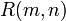is defined as the smallest positive integersuch that, for any graph whose vertex set has size, either the clique number is at leastor the independence number is at least. More explicitly, for any graph whose vertex set has size, the graph must either contain a-clique (i.e.,vertices all adjacent to each other) or an independent set of size(vertices no two of which are adjacent to each other).

## Facts

• Ramsey numbers are symmetric: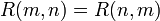•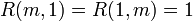for all positive integers•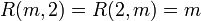for all positive integers• Recurrence relation bound for Ramsey numbers: This states that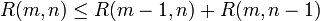. Moreover, if both the numbers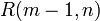and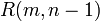are even, then we get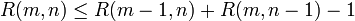• Binomial coefficient bound for Ramsey numbers: This states that## Particular cases(precise value if known) Proof that this is an upper bound Example graph with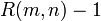vertices that does not have a-clique and does not have a-independent set
1 1 1
2 1 1
2 2 2
3 1 1
3 2 3
3 3 6 We use the recurrence relation bound for Ramsey numbers, to get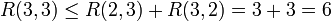cycle graph:C5
4 1 1
4 2 4
4 3 9 We use the recurrence relation bound for Ramsey numbers, to get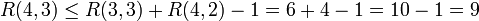. Note that we can subtract 1 because both numbers are even. cube graph
4 4 18 We use the recurrence relation bound for Ramsey numbers, to get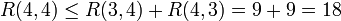Paley graph:P17